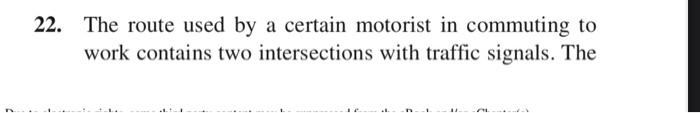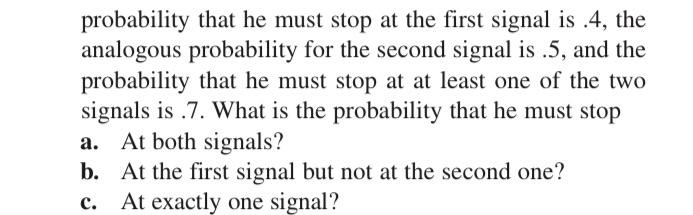# Question 22. The route used by a certain motorist in commuting to work contains two intersections with traffic signals. The probability that he must stop at the first signal is .4, the analogous probability for the second signal is.5, and the probability that he must stop at at least one of the two signals is 7. What is the probability that he must stop a. At both signals? b. At the first signal but not at the second one? At exactly one signal? c.OV4QFT The Asker · Probability and StatisticsTranscribed Image Text: 22. The route used by a certain motorist in commuting to work contains two intersections with traffic signals. The probability that he must stop at the first signal is .4, the analogous probability for the second signal is.5, and the probability that he must stop at at least one of the two signals is 7. What is the probability that he must stop a. At both signals? b. At the first signal but not at the second one? At exactly one signal? c.
More
Transcribed Image Text: 22. The route used by a certain motorist in commuting to work contains two intersections with traffic signals. The probability that he must stop at the first signal is .4, the analogous probability for the second signal is.5, and the probability that he must stop at at least one of the two signals is 7. What is the probability that he must stop a. At both signals? b. At the first signal but not at the second one? At exactly one signal? c.